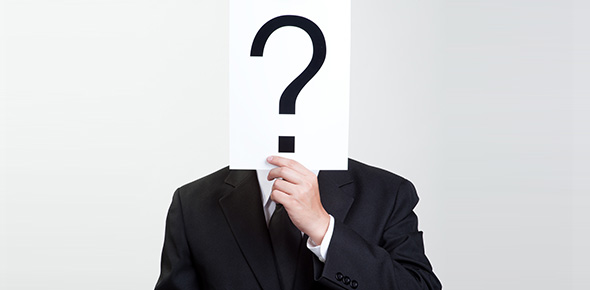# Early Secondary Mathematics Level 1 (M1) Placement Assessment

14 Questions | Total Attempts: 841Settings• 1.
Calculate the answer correct to 1 decimal place:
• 2.
Estimate the value of the following, correct to 2 significant figures:
• A.

4.1

• B.

4.2

• C.

4.3

• D.

4.4

• E.

4.5

• 3.
The ticket price to a movie is \$9.50 for each adult and \$4.75 for each child. Mrs. Hai took her children to watch a movie with her.  She paid a total of \$28.50 for the tickets. How many children did she take?
• A.

3

• B.

4

• C.

5

• D.

6

• E.

7

• 4.
Saira is twice as old as her sister Naira. Three years ago Saira was times as old as Naira. How old are Saira and Naira now?
• A.

Saira is 18 years old, Naira is 9 years old

• B.

Saira is 16 years old, Naira is 8 years old

• C.

Saira is 20 years old, Naira is 10 years old

• D.

Saira is 18 years old, Naira is 12 years old

• E.

Saira is 15 years old, Naira is 9 years old

• 5.
Two rectangles have the same length. The first rectangle's width is half its length, and the second rectangle's width is two-thirds its length. If the area of the larger rectangle is 10 cm square, what is the area of the smaller rectangle?
• A.

9.5 cm square

• B.

9 cm square

• C.

8.5 cm square

• D.

8 cm square

• E.

7.5 cm square

• 6.
A cylindrical barrel is 30 cm in radius and 70 cm in height. The barrel is half full of water. Water is poured into the barrel at the rate of 0.05 liters per second. How long will it take to fill the barrel with water? Note: 1 liter = 1000 cm cube
• A.

31.5 min

• B.

1860 sec

• C.

1980 min

• D.

33 min

• E.

34 min 30 sec

• 7.
A car drives from point A to point B in 3 hours and 45 minutes. The return journey takes 2 hours and 30 minutes. If the average speed for the whole journey is 40 km/h what is: (a) the distance between point A and B? (b) the average speed for the return journey (point B to point A) only?
• A.

(a) 110 km (b) 50 km/h

• B.

(a) 125 km (b) 50 km/h

• C.

(a) 250 km (b) 60 km/h

• D.

(a) 180 km (b) 55 km/h

• E.

(a) 250 km (b) 50 km/h

• 8.
Jamal goes to the market to buy apples, bananas and carrots. He buys 20% more bananas than apples, and one-third less carrots than bananas. If the number of apples he bought was 15, how many carrots did he buy?
• A.

10 carrots

• B.

12 carrots

• C.

14 carrots

• D.

15 carrots

• E.

18 carrots

• 9.
(a) Calculate the value of y when x is 6 (b) Calculate the value of x when y is (c) If the line made by the equation is plotted on graph paper, which way does it slope?
• A.

(a) 8 (b) 4 (c) upward sloping

• B.

(a) 9 (b) 4 (c) downward sloping

• C.

(a) 11 (b) 4 (c) downward sloping

• D.

(a) 11 (b) 4 (c) upward sloping

• E.

(a) 11 (b) 5 (c) downward sloping

• 10.
The table below shows the marks scored by 90 students on a Maths test. (a) What percentage of students scored 70% or less? (b) If a pie chart were to show students who scored 80% and above, approximately what would be the angle of the sector that represents these students?
• A.

(a) 45% (b) 90 degrees

• B.

(a) 50% (b) 90 degrees

• C.

(a) 45% (b) 25 degrees

• D.

(a) 50% (b) 25 degrees

• E.

(a) 50% (b) 75 degrees

• 11.
In the figure, BCD is a straight line and ABC is an equilateral triangle.Find
• A.

35 degrees

• B.

25 degrees

• C.

45 degrees

• D.

30 degrees

• E.

17.5 degrees

• 12.
Find the unknown angles x, y and z in the figure below.
• A.

X=52 degrees, y=31 degrees, z=97 degrees

• B.

X=48 degrees, y=32 degrees, z=100 degrees

• C.

X=48 degrees, y=30 degrees, z=102 degrees

• D.

X=50 degrees, y=31 degrees, z=99 degrees

• E.

X=52 degrees, y=32 degrees, z=96 degrees